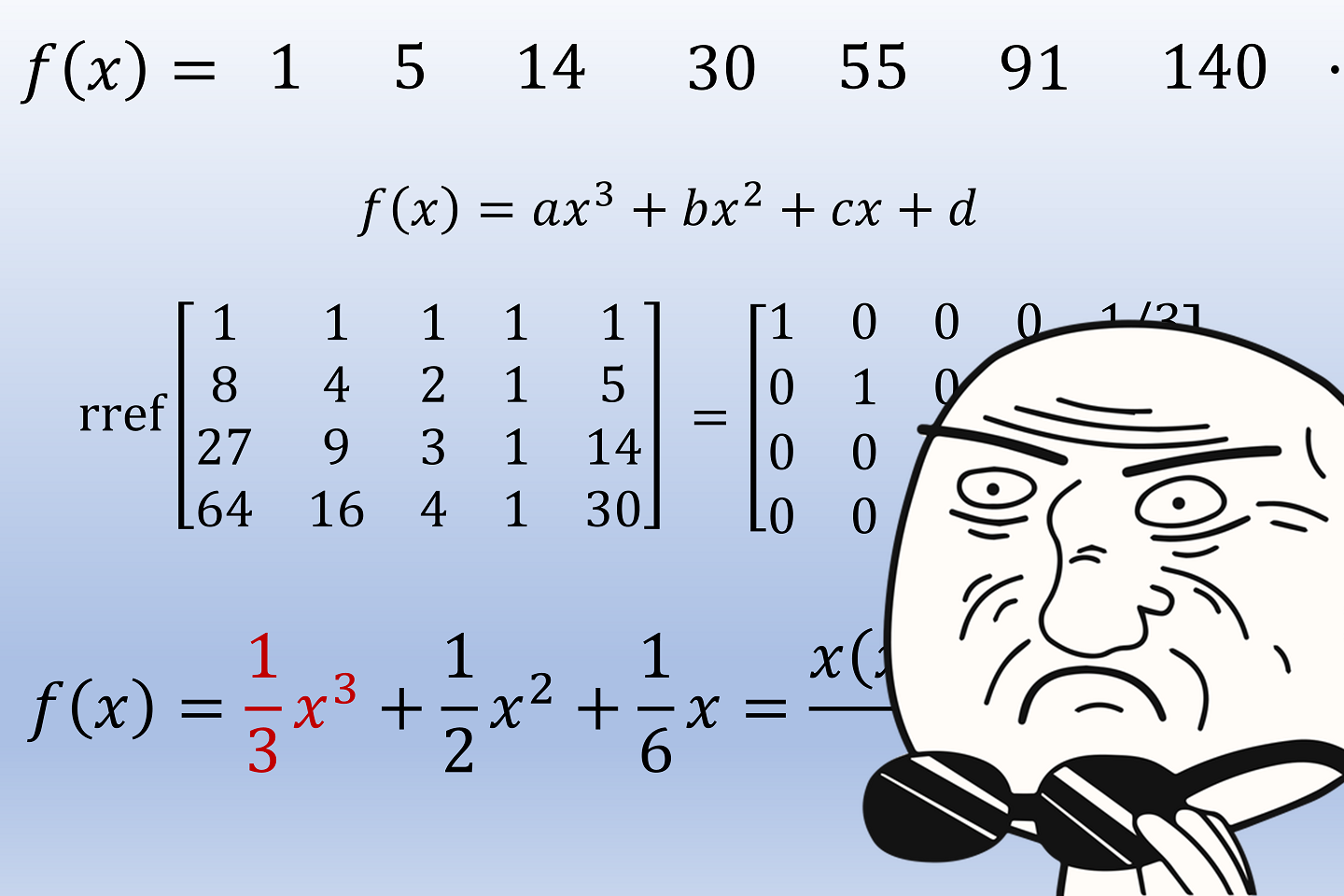# Discreet Discrete Calculus

October 21, 2017
Mathematics
Teaching

Over the past week, I went to the Georgia Mathematics Conference (GMC) at Rock Eagle, held by the Georgia Council of Teachers of Mathematics (GCTM).  The GMC is one of the events I look forward to most every year --- tons of math educators and advocates sharing lessons, techniques, and ideas about how to best teach math to students from kindergarten through college.  I always enjoy sharing my own perspectives as well (even when they do get a bit bizarre!)

This time, I got to share the results of a lesson that I guinea-pigged on my Honors Precalculus class last year, where they explored the relationships between polynomial sequences, common differences, and partial sums.  The presentation from the GMC uses the techniques we looked at to develop the formula for the sum of the first $$n$$ perfect squares:

$1^2+2^2+3^2+\cdots+n^2=\frac{n(n+1)(2n+1)}{6}$

At the GMC, we did go a bit further than my class did --- they didn't do the full development of discrete calculus --- but some knowledge of where the ideas lead is never a bad thing, and a different class at a different school may even be able to go further!

Here is the PowerPoint from the presentation.  If you find it useful, or have any questions, please don't hesitate to leave a comment!